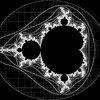## Lissajous XXV• Available
Products
44
• Artist
Notes

## Artist's Description

// Created with Processing
// Draws a Lissajous figures
// 4 April 2011
// Examples

int screen_width = 2000;
int screen_height = 2000;

/* Scale image and reduce by 5 pixels to remove from edge of screen */
int AX = (screen_width / 2) – 5;
int BX = (screen_height / 2) – 5;

float a = 10;
float b = 2;
float x1, y1, x2, y2;

int counter = 0;
int saveCounter = 0;
String file_name;
//
void setup(){
smooth();
fill(255);
strokeWeight(2);
size(screen_width, screen_height);
}
//
void draw(){
fill(#000000);
//
x1 = (sin(a * counter) * AX) + screen_width / 2;
//
y1 = (sin(b * counter) * BX) + screen_height / 2;
//
counter = counter + 1;
//
y2 = screen_width / 2;
//
x2 = screen_height / 2;
//
line(x1,y1,x2,y2);
//
ellipse((sin(a * counter) * AX) + screen_width / 2 , (sin(b * counter) * BX) + screen_height / 2, 10, 10);
//
saveCounter = saveCounter + 1;
if (saveCounter == 364) {
String file_name = “Lissajou_a=” + str(a) + “_b=” + str(b);
save(file_name);
noLoop();
}
}